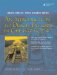# Derivation with Polymorphism

We can now discuss a very powerful feature of object-oriented programming: polymorphism. Example 6.6 differs from the previous example in only one important way: the use of the keyword virtual in the base class definition.

Example 6.6. src/derivation/qpoly/student.h

 ```[ . . . . ] class Student { public: Student(QString nm, long id, QString m_Major, int year = 1); virtual QString getClassName() const; <-- 1 QString toString() const; <-- 2 virtual ~Student() {} <-- 3 QString yearStr() const; private: QString m_Name; QString m_Major; long m_StudentId; protected: int m_Year; }; // derived classes are the same as before... [ . . . . ] ```   (1)Note the keyword virtual here. (2)This should be virtual, too. (3)It is a good idea to make the destructor virtual, too.

By simply adding the keyword virtual to at least one member function we have created a polymorphic type. When virtual is specified on a function, it becomes a method in that class and all derived classes. Example 6.7 shows the same client code again:

Example 6.7. src/derivation/qpoly/student-test.cpp

 ```#include #include "student.h" static QTextStream cout(stdout, QIODevice::WriteOnly); void graduate(Student* student) { cout << " The following " << student->getClassName() << " has graduated " << student->toString() << " "; } int main() { Undergrad us("Frodo", 5562, "Ring Theory", 4); GradStudent gs("Bilbo", 3029, "History", 6, GradStudent::fellowship); cout << "Here is the data for the two students: "; cout << gs.toString() << endl; cout << us.toString() << endl; cout << " Here is what happens when they graduate: "; graduate(&us); graduate(&gs); return 0; } ```

Running this version of the program produces slightly different output, as shown here.

```Here is the data for the two students:
History
[Support: fellowship ]

[Undergrad] name: Frodo Id: 5562 Year: senior Major: Ring Theory

Here is what happens when they graduate:

[Undergrad] name: Frodo Id: 5562 Year: senior Major: Ring Theory

History
```

 What happened to the Fellowship?

Notice that we now see [GradStudent] and [UnderGrad] in the output, thanks to the fact that getClassName() is virtual . There is still a problem with the output of graduate() for the GradStudent, however. The Support piece is missing.

With polymorphism, indirect calls (via pointers and references) to methods are resolved at runtime. This is sometimes called dynamic, or late run-time binding. Direct calls (not through pointers or references) of methods are still resolvable by the compiler. That is called static binding or compile-time binding.

In this example, when graduate() receives the address of a GradStudent object, student->toString() calls the Student version of the function. However, when the Student::toString() calls getClassName() (indirectly through this, a base class pointer), it is a virtual method call, bound at runtime.

Try adding the keyword virtual to the declaration of toString() in the Student class definition so that you can see the support data displayed properly.

In C++, dynamic binding is an option that one must switch on with the keyword virtual.

Exercise: Derivation with Polymorphism

Be the computer and predict the output of the programs shown in Examples 6.8 through 6.12. Then compile and run the programs to check your answers.

1.

Example 6.8. src/polymorphic1.cc

 ```#include using namespace std; class A { public: virtual void foo() { cout << "A's foo()" << endl; bar(); } virtual void bar() { cout << "A's bar()" << endl; } }; class B: public A { public: void foo() { cout << "B's foo()" << endl; A::foo(); } void bar() { cout << "B's bar()" << endl; } }; int main() { B bobj; A *aptr = &bobj; aptr->foo(); A aobj = *aptr; aobj.foo(); } ```
2.

Example 6.9. src/polymorphic2.cc

 ```#include using namespace std; class A { public: virtual void foo() { cout << "A's foo()" << endl; } }; class B: public A { public: void foo() { cout << "B's foo()" << endl; } }; class C: public B { public: void foo() { cout << "C's foo()" << endl; } }; int main() { C cobj; B *bptr = &cobj; bptr->foo(); A* aptr = &cobj; aptr->foo(); } ```
3.

Example 6.10. src/derivation/exercise/Base.h

 ```[ . . . . ] class Base { public: Base(); void a(); virtual void b() ; virtual void c(bool condition=true); virtual ~Base() {} }; class Derived : public Base { public: Derived(); virtual void a(); void b(); void c(); }; [ . . . . ] ```

Example 6.11. src/derivation/exercise/Base.cpp

 ```[ . . . . ] Base::Base() { cout << "Base::Base()" << endl; a(); c(); } void Base::c(bool condition) { cout << "Base::c()" << endl; } void Base::a() { cout << "Base::A" << endl; b(); } void Base::b() { cout << "Base::B" << endl; } Derived::Derived() { cout << "Derived::Derived() " << endl; } void Derived::a() { cout << "Derived::a()" << endl; c(); } void Derived::b() { cout << "Derived::b()" << endl; } void Derived::c() { cout << "Derived::c()" << endl; } [ . . . . ] ```

Example 6.12. src/derivation/exercise/main.cpp

 ```[ . . . . ] int main (int argc, char** argv) { Base b; Derived d; cout << "Objects Created" << endl; b.b(); cout << "Calling derived methods" << endl; d.a(); d.b(); d.c(); cout << ".. via base class pointers..." << endl; Base* bp = &d; bp->a(); bp->b(); bp->c(); } [ . . . . ] ```

### Derivation from an Abstract Base ClassAn Introduction to Design Patterns in C++ with Qt 4
ISBN: 0131879057
EAN: 2147483647
Year: 2004
Pages: 268